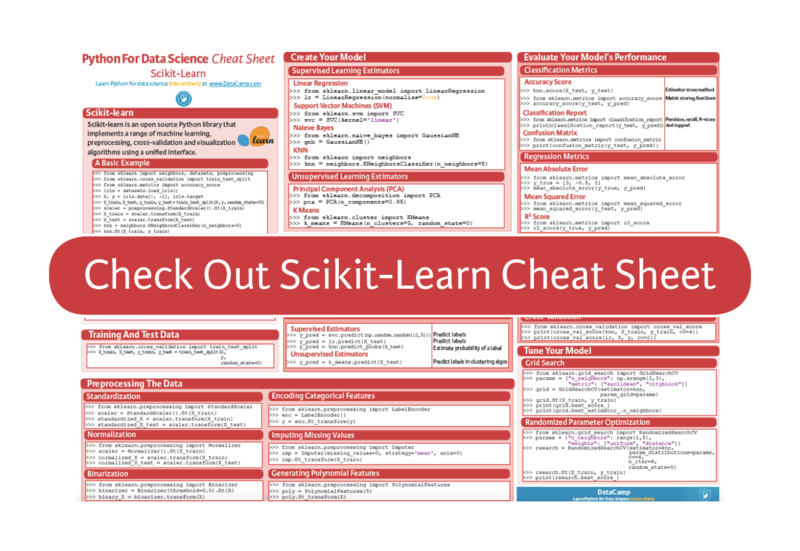Official Blog
python
+1

# Scikit-Learn Cheat Sheet: Python Machine Learning

A handy scikit-learn cheat sheet to machine learning with Python, including code examples.

Most of you who are learning data science with Python will have definitely heard already about scikit-learn, the open source Python library that implements a wide variety of machine learning, preprocessing, cross-validation and visualization algorithms with the help of a unified interface.

If you're still quite new to the field, you should be aware that machine learning, and thus also this Python library, belong to the must-knows for every aspiring data scientist.

That's why DataCamp has created a scikit-learn cheat sheet for those of you who have already started learning about the Python package, but that still want a handy reference sheet. Or, if you still have no idea about how scikit-learn works, this machine learning cheat sheet might come in handy to get a quick first idea of the basics that you need to know to get started.

Either way, we're sure that you're going to find it useful when you're tackling machine learning problems!

This scikit-learn cheat sheet will introduce you to the basic steps that you need to go through to implement machine learning algorithms successfully: you'll see how to load in your data, how to preprocess it, how to create your own model to which you can fit your data and predict target labels, how to validate your model and how to tune it further to improve its performance.In short, this cheat sheet will kickstart your data science projects: with the help of code examples, you'll have created, validated and tuned your machine learning models in no time.

So what are you waiting for? Time to get started!

## Python For Data Science Cheat Sheet: Scikit-learn

Scikit-learn is an open source Python library that implements a range of machine learning, preprocessing, cross-validation and visualization algorithms using a unified interface.

### A Basic Example

>>> from sklearn import neighbors, datasets, preprocessing
>>> from sklearn.model_selection import train_test_split
>>> from sklearn.metrics import accuracy_score
>>> X, y = iris.data[:, :2], iris.target
>>> X_train, X_test, y_train, y_test = train_test_split(X, y, random_state=33)
>>> scaler = preprocessing.StandardScaler().fit(X_train)
>>> X_train = scaler.transform(X_train)
>>> X_test = scaler.transform(X_test)
>>> knn = neighbors.KNeighborsClassifier(n_neighbors=5)
>>> knn.fit(X_train, y_train)
>>> y_pred = knn.predict(X_test)
>>> accuracy_score(y_test, y_pred)

Your data needs to be numeric and stored as NumPy arrays or SciPy sparse matrices. Other types that are convertible to numeric arrays, such as Pandas DataFrame, are also acceptable.

>>> import numpy as np
>>> X = np.random.random((10,5))
>>> y = np.array(['M','M','F','F','M','F','M','M','F','F','F'])
>>> X[X < 0.7] = 0

### Preprocessing The Data

#### Standardization

>>> from sklearn.preprocessing import StandardScaler
>>> scaler = StandardScaler().fit(X_train)
>>> standardized_X = scaler.transform(X_train)
>>> standardized_X_test = scaler.transform(X_test)

#### Normalization

>>> from sklearn.preprocessing import Normalizer
>>> scaler = Normalizer().fit(X_train)
>>> normalized_X = scaler.transform(X_train)
>>> normalized_X_test = scaler.transform(X_test)

#### Binarization

>>> from sklearn.preprocessing import Binarizer
>>> binarizer = Binarizer(threshold=0.0).fit(X)
>>> binary_X = binarizer.transform(X)

#### Encoding Categorical Features

>>> from sklearn.preprocessing import LabelEncoder
>>> enc = LabelEncoder()
>>> y = enc.fit_transform(y)

#### Imputing Missing Values

>>>from sklearn.preprocessing import Imputer
>>>imp = Imputer(missing_values=0, strategy='mean', axis=0)
>>>imp.fit_transform(X_train)

#### Generating Polynomial Features

>>> from sklearn.preprocessing import PolynomialFeatures
>>> poly = PolynomialFeatures(5)
>>> oly.fit_transform(X)

### Training And Test Data

>>> from sklearn.model_selection import train_test_split
>>> X_train, X_test, y_train, y_test = train_test_split(X,y,random_state=0)

#### Supervised Learning Estimators

Linear Regression

>>> from sklearn.linear_model import LinearRegression
>>> lr = LinearRegression(normalize=True)

Support Vector Machines (SVM)

>>> from sklearn.svm import SVC
>>> svc = SVC(kernel='linear')

Naive Bayes

>>> from sklearn.naive_bayes import GaussianNB
>>> gnb = GaussianNB()

KNN

>>> from sklearn import neighbors
>>> knn = neighbors.KNeighborsClassifier(n_neighbors=5)

Unsupervised Learning Estimators

Principal Component Analysis (PCA)

>>> from sklearn.decomposition import PCA
>>> pca = PCA(n_components=0.95)

K Means

>>> from sklearn.cluster import KMeans
>>> k_means = KMeans(n_clusters=3, random_state=0)

### Model Fitting

#### Supervised learning

>>> lr.fit(X, y)
>>> knn.fit(X_train, y_train)
>>> svc.fit(X_train, y_train)
Unsupervised Learning
>>> k_means.fit(X_train)
>>> pca_model = pca.fit_transform(X_train)

### Prediction

Supervised Estimators

>>> y_pred = svc.predict(np.random.random((2,5)))
>>> y_pred = lr.predict(X_test)
>>> y_pred = knn.predict_proba(X_test))

Unsupervised Estimators

>>> y_pred = k_means.predict(X_test)

#### Classification Metrics

Accuracy Score

>>> knn.score(X_test, y_test)
>>> from sklearn.metrics import accuracy_score
>>> accuracy_score(y_test, y_pred)

Classification Report

>>> from sklearn.metrics import classification_report
>>> print(classification_report(y_test, y_pred)))

Confusion Matrix

>>> from sklearn.metrics import confusion_matrix
>>> print(confusion_matrix(y_test, y_pred)))

#### Regression Metrics

Mean Absolute Error

>>> from sklearn.metrics import mean_absolute_error
>>> y_true = [3, -0.5, 2])
>>> mean_absolute_error(y_true, y_pred))

Mean Squared Error

>>> from sklearn.metrics import mean_squared_error
>>> mean_squared_error(y_test, y_pred))

R2 Score

>>> from sklearn.metrics import r2_score
>>> r2_score(y_true, y_pred))

#### Clustering Metrics

>>> from sklearn.metrics import adjusted_rand_score
>>> adjusted_rand_score(y_true, y_pred))

Homogeneity

>>> from sklearn.metrics import homogeneity_score
>>> homogeneity_score(y_true, y_pred))

V-measure

>>> from sklearn.metrics import v_measure_score
>>> metrics.v_measure_score(y_true, y_pred))

#### Cross-Validation

>>> print(cross_val_score(knn, X_train, y_train, cv=4))
>>> print(cross_val_score(lr, X, y, cv=2))

#### Grid Search

>>> from sklearn.grid_search import GridSearchCV
>>> params = {"n_neighbors": np.arange(1,3), "metric": ["euclidean", "cityblock"]}
>>> grid = GridSearchCV(estimator=knn,param_grid=params)
>>> grid.fit(X_train, y_train)
>>> print(grid.best_score_)
>>> print(grid.best_estimator_.n_neighbors)

#### Randomized Parameter Optimization

>>> from sklearn.grid_search import RandomizedSearchCV
>>> params = {"n_neighbors": range(1,5), "weights": ["uniform", "distance"]}
>>> rsearch = RandomizedSearchCV(estimator=knn,
param_distributions=params,
cv=4,
n_iter=8,
random_state=5)
>>> rsearch.fit(X_train, y_train)
>>> print(rsearch.best_score_)

### Going Further

Begin with our scikit-learn tutorial for beginners, in which you'll learn in an easy, step-by-step way how to explore handwritten digits data, how to create a model for it, how to fit your data to your model and how to predict target values. In addition, you'll make use of Python's data visualization library matplotlib to visualize your results.

PS. Don't miss our Bokeh cheat sheet, the Pandas cheat sheet or the Python cheat sheet for data science